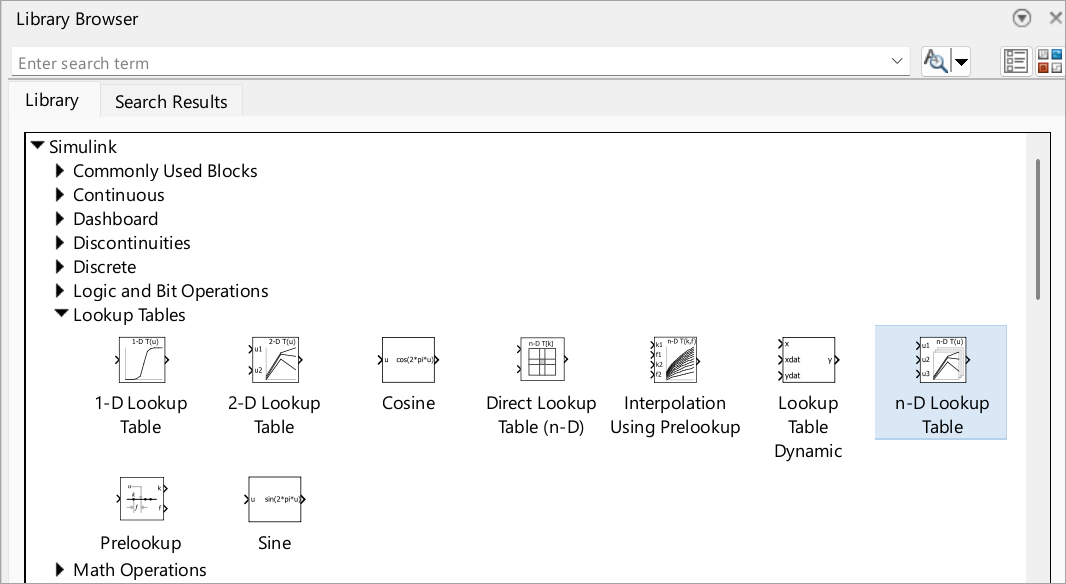Nonlinearity

Model nonlinearity with lookup tables, approximate mathematical functions by mapping input values to output values

If you are new to modeling nonlinearity, first try using a lookup table block. Lookup table blocks use arrays of data to map input values to output values, approximating mathematical functions. To approximate a function in N variables, use the n-D Lookup Table block:Blocks

 1-D Lookup Table Approximate one-dimensional function 2-D Lookup Table Approximate two-dimensional function Direct Lookup Table (n-D) Index into n-dimensional table to retrieve element, vector, or 2-D matrix Interpolation Using Prelookup Use precalculated index and fraction values to accelerate approximation of N-dimensional function Lookup Table Dynamic Approximate a one-dimensional function using dynamic table n-D Lookup Table Approximate n-dimensional function Prelookup Compute index and fraction for Interpolation Using Prelookup block Sine, Cosine Implement fixed-point sine or cosine wave using lookup table approach that exploits quarter wave symmetry

Classes

 Simulink.LookupTable Store and share lookup table and breakpoint data, configure the data for ASAP2 and AUTOSAR code generation Simulink.Breakpoint Store and share data for a breakpoint set, configure the data for ASAP2 and AUTOSAR code generation Simulink.lookuptable.Evenspacing Configure even spacing set data for lookup table object Simulink.lookuptable.Table Configure table data for lookup table object Simulink.lookuptable.Breakpoint Configure breakpoint set data for lookup table object Simulink.lookuptable.StructTypeInfo Configure settings for structure type that lookup table object uses in the generated code

Examples and How To

Enter Breakpoints and Table Data

Specify breakpoint data sets and table data for lookup table blocks.

Edit Lookup Tables

Change the elements of lookup tables.

Import Lookup Table Data from MATLAB

You can import table and breakpoint data from variables in the MATLAB workspace by referencing them in the Table and Breakpoints tab of the dialog box.

Import Lookup Table Data from Excel

Import data into a lookup table with the xlsread function.

Create a Logarithm Lookup Table

Work with lookup table blocks.

Prelookup and Interpolation Blocks

Use Prelookup and Interpolation Using Prelookup blocks together.

Update Lookup Table Blocks to New Versions

Update existing models to use current versions of lookup table blocks.

Optimize Generated Code for Lookup Table Blocks

Optimize generated code for lookup table blocks.

View Lookup Table Data

Work with lookup table objects with a lookup table viewer.

Concepts

A lookup table block uses an array of data to map input values to output values, approximating a mathematical function.

Anatomy of a Lookup Table

Breakpoint data sets and table data are components of a lookup table.

Lookup Tables Block Library

Choose among the various blocks in the Lookup Tables library.

Guidelines for Choosing a Lookup Table

Select the best lookup table for your application.

Characteristics of Lookup Table Data

Comply with lookup table data requirements and represent discontinuous data.

Methods for Approximating Function Values

Set the method by which lookup table blocks approximate function values.